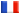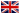# Séminaire de Cryptographie

## Rolnald Cramer### Blackbox Secret Sharing from Primitive Sets in Algebraic Number Fields

A {\em black-box} secret sharing scheme (BBSSS) for a given access structure works in exactly the same way over any finite Abelian group, as it only requires black-box access to group operations and to random group elements. In particular, there is no dependence on e.g.\ the structure of the group or its order. The expansion factor of a BBSSS is the length of a vector of shares (the number of group elements in it) divided by the number of players $n$.

In 2002 Cramer and Fehr proposed a threshold BBSSS with an asymptotically minimal expansion factor $\Theta(\log n)$.

We present a BBSSS that is based on a new paradigm, namely, {\em primitive sets in algebraic number fields}. This leads to a new BBSSS with an expansion factor that is absolutely minimal up to an additive term of at most~2, which is an improvement by a constant additive factor. The construction uses techniques from algebraic number theory as well as algebraic geometry.

We provide good evidence that our scheme is considerably more efficient in terms of the computational resources it requires. Indeed, the number of group operations to be performed is $\tilde{O}(n^2)$ instead of $\tilde{O}(n^3)$ for sharing and $\tilde{O}(n^{1.6})$ instead of $\tilde{O}(n^{2.6})$ for reconstruction. Finally, we show that our scheme, as well as that of Cramer and Fehr, has asymptotically optimal randomness efficiency.

This is talk is based on joint work with Serge Fehr, Hendrik Lenstra, and Martijn Stam. An article with these results appears in the Proceedings of the 25th Annual IACR CRYPTO Conference, 2005.